In :
%matplotlib inline
from IPython.html.widgets import interact
from scipy import  stats
import seaborn as sns
import pandas as pd


Previously, we talk about maximum likelihood estimation and maximum a-posteriori estimation and in each case we started out with a probability density function of some kind and we further assumed that the samples were identically distributed and independent. The idea behind robust statistics is to construct estimators that can survive the weakening of either or both of these assumptions.

The first idea to consider is the notion of location, which is a generalization of the idea of "central value". Typically, we just use an estimate of the mean for this, but we will see shortly why that is a bad idea. The general idea of Location satisfies the following requirements

Let $X$ be a random variable with distribution $F$, and let $\theta(X)$ be some descriptive measure of $F$. Then $\theta(X)$ is said to be a measure of location if for any constants a and b, we have the following:

$$\theta(X+b) = \theta(X) +b$$$$\theta(-X) = -\theta(X)$$$$X \ge 0 \Rightarrow \theta(X) \ge 0$$$$\theta(a X) = a\theta(X)$$

The first condition is called location equivariance (or shift-invariance in signal processing lingo). The fourth condition is called scale equivariance, which means that the units that $X$ is measured in should not effect the value of the location estimator. These Requirements capture the idea of what we intuitively mean by centrality of a distribution, or where most of the probability mass is located.

For example, the mean estimator is $\hat{\mu}=\frac{1}{n}\sum X_i$. The first requirement is obviously satisfied as $\hat{\mu}=\frac{1}{n}\sum (X_i+b) = b + \frac{1}{n}\sum X_i =b+\hat{\mu}$. Let us consider the second requirement:$\hat{\mu}=\frac{1}{n}\sum -X_i = -\hat{\mu}$. Finally, the last requirement is satisfied with $\hat{\mu}=\frac{1}{n}\sum a X_i =a \hat{\mu}$.

## What do we mean by robust estimators?¶

Now that we have the generalized location of centrality embodied in the location parameter, what can we do with it? The next idea is to nail down is the concept of robust estimators. Previously, we assumed that our samples were all identically distributed. The key idea is that the samples might be actually coming from a distribution that is contaminated by another nearby distribution, as in the following:

$$F(X) = \epsilon G(X) + (1-\epsilon)H(X)$$

where $\epsilon$ is between zero and one. This means that our data samples $\lbrace X_i \rbrace$ actually derived from two separate distributions, $G(X)$ and $H(X)$. We just don't know how they are mixed together. What we really want is an estimator that captures the location of $G(X)$ in the face of random intermittent contamination by $H(X)$. It can get even worse than that because we don't know that there is only one contaminating $H(X)$ distribution out there. There may be a whole family of distributions that are contaminating $G(X)$ that we don't know of. This means that whatever estimators we construct have to be derived from families of distributions instead of a distribution, which is what we have been assuming for maximum-likelihood estimators. This is what makes robust estimation so difficult --- the extended theory has to deal with spaces of function distributions instead of particular parameters of a particular probability distribution.

• Influence function
• Outlier Detection
• Estimates of location
• definition of location
• Trimmed means
• Windsorized means
• Hodges Lehmann statistics
• Asymptotic efficiency
• Fisher Consistent

• Robust Regression

- least median
- outliers
In :
n0=stats.norm(0,1)
n1=stats.norm(1,2)
xi = linspace(-5,5,100)

fig,ax=subplots()
ax.plot(xi,n0.pdf(xi))
ax.plot(xi,n1.pdf(xi))

def bias_coin(phead = .5):
while True:
yield int( np.random.rand() < phead )

pct_mixed  = 0.1
bias_coin_gen = bias_coin(pct_mixed)
dual_set = [n0,n1]
samples  = [ dual_set[bias_coin_gen.next()].rvs() for i in range(500) ]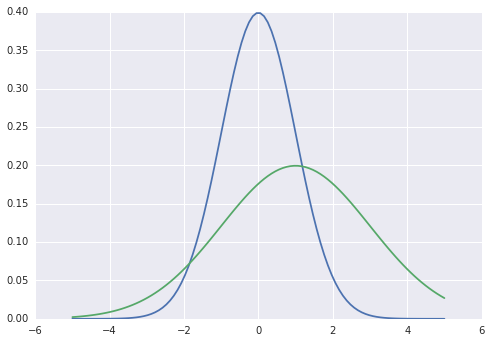In :
hist(samples,bins=20)
title('average = %3.3f, median=%3.3f pct_mixed=%3.3f'%(mean(samples),np.median(samples),pct_mixed))

Out:
<matplotlib.text.Text at 0x118ca270>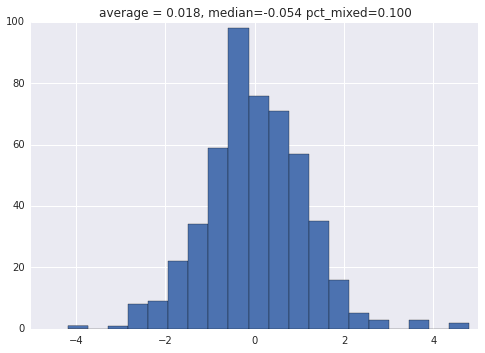In :
import sympy.stats
from sympy.abc import x
eps = sympy.symbols('epsilon')

mixed_cdf = sympy.stats.cdf(sympy.stats.Normal('x',0,1),'x')(x)*(1-eps) + eps*sympy.stats.cdf(sympy.stats.Normal('x',1,2),'x')(x)
mixed_pdf = sympy.diff(mixed_cdf,x)

In :
def plot_mixed_dist(epsilon=.1):
n1 = stats.norm(1,2)
xi = linspace(-5,5,100)
fig,ax = subplots()
ax.plot(xi,[sympy.lambdify(x,mixed_pdf.subs(eps,epsilon))(i) for i in xi],label='mixed',lw=2)
ax.plot(xi,n0.pdf(xi),label='g(x)',linestyle='--')
ax.plot(xi,n1.pdf(xi),label='h(x)',linestyle='--')
ax.legend(loc=0)
ax.set_title('epsilon = %2.2f'%(epsilon))
ax.vlines(0,0,.4,linestyle='-',color='g')
ax.vlines(epsilon,0,.4,linestyle='-',color='b')

interact(plot_mixed_dist,epsilon=(0,1,.05))

Out:
<function __main__.plot_mixed_dist>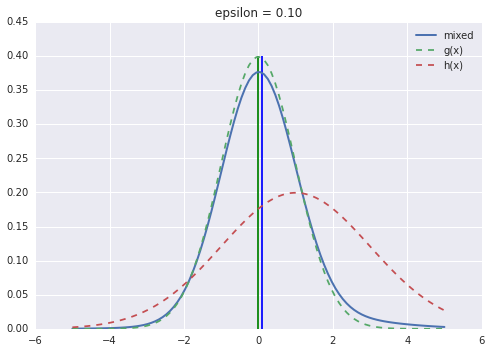## M-estimators¶

M-estimators are generalized maximum likelihood estimators. Recall that for maximum likelihood, we want to maximize the likelihood function as in the following:

$$L_{\mu}(x_i) = \prod f_0(x_i-\mu)$$

and then to find the estimator $\hat{\mu}$ so that

$$\hat{\mu} = \arg \max_{\mu} L_{\mu}(x_i)$$

So far, everything is the same as our usual maximum-likelihood derivation except for the fact that we don't know $f_0$, the distribution of the $\lbrace X_i\rbrace$. Making the convenient definition of

$$\rho = -\log f_0$$

we obtain the more convenient form of the likelihood product and the optimal $\hat{\mu}$ as

$$\hat{\mu} = \arg \min_{\mu} \sum \rho(x_i-\mu)$$

If $\rho$ is differentiable, then differentiating this with respect to $\mu$ gives

$$\sum \psi(x_i-\hat{\mu}) = 0$$

with $\psi = \rho'$ and for technical reasons we will assume that $\psi$ is increasing. The key idea here is we want to consider general $\rho$ functions that my not be MLE for any distribution.

### The distribution of M-estimates¶

For a given distribution $F$, we define $\mu_0=\mu(F)$ as the solution to the following

$$\mathbb{E}_F(\psi(x-\mu_0))= 0$$

It is technical to show, but it turns out that $\hat{\mu} \sim \mathcal{N}(\mu_0,\frac{v}{n})$ with

$$v = \frac{\mathbb{E}_F(\psi(x-\mu_0)^2)}{(\mathbb{E}_F(\psi^\prime(x-\mu_0)))^2}$$

Thus, we can say that $\hat{\mu}$ is asymptotically normal with asymptotic value $\mu_0$ and asymptotic variance $v$. This leads to the efficiency ratio which is defined as the following:

$$\texttt{Eff}(\hat{\mu})= \frac{v_0}{v}$$

where $v_0$ is the asymptotic variance of the MLE and measures how near $\hat{\mu}$ is to the optimum. for example, if for two estimates with asymptotic variances $v_1$ and $v_2$, we have $v_1=3v_2$, then first estimate requires three times as many observations to obtain the same variance as the second.

For example, for the sample mean (i.e. $\hat{\mu}=\frac{1}{n} \sum X_i$) with $F=\mathcal{N}$, we have $\rho=x^2/2$ and $\psi=x$ and also $\psi'=1$. Thus, we have $v=\mathbb{V}(x)$. Alternatively, using the sample median as the estimator for the location, we have $v=\frac{1}{4 f(\mu_0)^2}$. Thus, if we have $F=\mathcal{N}(0,1)$, for the sample median, we obtain $v=\frac{2\pi}{4} \approx 1.571$. This means that the sample median takes approximately 1.6 times as many samples to obtain the same variance for the location as the sample mean.

One way to think about M-estimates is a weighted means. Most of the time, we have $\psi(0)=0$ and $\psi'(0)$ exists so that $\psi$ is approximately linear at the origin. Using the following definition:

$$W(x) = \begin{cases} \psi(x)/x & \text{if} \: x \neq 0 \\ \psi'(x) & \text{if} \: x =0 \end{cases}$$

We can write our earlier equation as follows:

$$\sum W(x_i-\hat{\mu})(x_i-\hat{\mu}) = 0$$

Solving this for $\hat{\mu}$ yields the following,

$$\hat{\mu} = \frac{\sum w_{i} x_i}{\sum w_{i}}$$

where $w_{i}=W(x_i-\hat{\mu})$. The question that remains is how to pick the $\psi$ functions.

### Huber functions¶

The family of Huber function is defined by the following:

$$\rho_k(x ) = \begin{cases} x^2 & \text{if} \: |x|\le k \\ 2 k |x|-k^2 & \text{if} \: |x| \gt k \end{cases}$$

with corresponding derivatives $2\psi_k(x)$ with

$$\psi_k(x ) = \begin{cases} x & \text{if} \: |x|\le k \\ \text{sgn}(x)k & \text{if} \: |x| \gt k \end{cases}$$

where the limiting cases $k \rightarrow \infty$ and $k \rightarrow 0$ correspond to the mean and median, respectively. To see this, take $\psi_{\infty} = x$ and therefore $W(x) = 1$ and thus the defining equation results in

$$\sum_{i=1}^{n} (x_i-\hat{\mu}) = 0$$

and then solving this leads to $\hat{\mu} = \frac{1}{n}\sum x_i$. Note that choosing $k=0$ leads to the sample median, but that is not so straightforward to solve for.

In :
fig,ax=subplots()
colors=['b','r']
for k in [1,2]:
ax.axis(ymax=2.3,ymin=-2.3)
ax.set_ylabel(r'$\psi(x)$',fontsize=28)
ax.set_xlabel(r'$x$',fontsize=24)
ax.legend(loc='best')
ax.set_title('Huber functions')
ax.grid()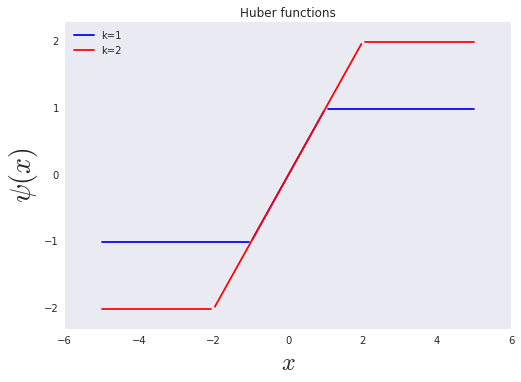The $W$ function corresponding to Huber's $\psi$ is the following:

$$W_k(x) = \min\Big{\lbrace} 1, \frac{k}{|x|} \Big{\rbrace}$$

which is plotted in the following cell for a few values of $k$.

In :
fig,ax=subplots()
ax.plot(xi,np.vstack([np.ones(xi.shape),2/abs(xi)]).min(axis=0),label='k=2')
ax.plot(xi,np.vstack([np.ones(xi.shape),1/abs(xi)]).min(axis=0),label='k=1')
ax.axis(ymax=1.1)
ax.legend(loc=0)
ax.set_title("Huber's weight function")

Out:
<matplotlib.text.Text at 0x12dd82d0>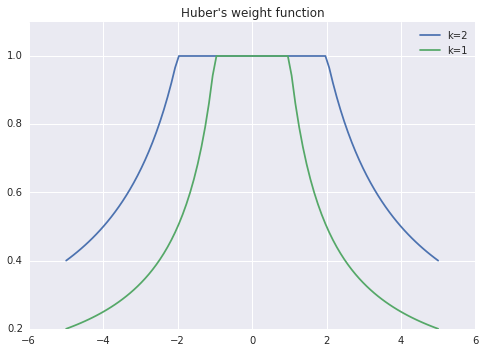Another alternative intuitive way to interpret the M-estimate is to rewrite the following:

$$\hat{\mu} = \hat{\mu} +\frac{1}{n}\sum_i \psi(x_i-\hat{\mu}) = \frac{1}{n} \sum_i \zeta(x_i,\hat{\mu})$$

which for the Huber family of functions takes on the form :

$$\zeta(x,\mu) = \begin{cases} \mu - k & \text{if} \: x \lt \mu-k \\ x & \text{if} \: \mu-k \le x \le \mu+k \\ \mu+k & \text{if} \: x \gt \mu \\ \end{cases}$$

Thus, the interpretation here is that $\hat{\mu}$ is the average of the truncated pseudo-observations $\zeta_i$ where the observations beyond a certain point are clipped at the $k$-offset of the $\hat{\mu}$.

In :
kvals= [.001,0.3,0.5,.7,1.00001,1.4,1.7,2,3,4,5]

In :
import pythonica
mma=pythonica.Pythonica()
mma.plot_dir='.'

In :
def closure_variance(mn=(0,1),std=(1,1)):
# close over specific F-distribution and integral terms
mma.eval('Clear["*"]') # clear workspace
mma.eval('lpdf=D[CDF[NormalDistribution[%g,%g], x](1-Epsilon)+Epsilon*CDF[NormalDistribution[%g,%g],x],x]'%(mn,std,mn,std))
denom=mma.eval('Integrate[lpdf,{x,-k,k},Assumptions -> k > 0]^2')
numer=mma.eval('Integrate[lpdf*Piecewise[{{x, Abs[x] < k},{k*Sign[x],Abs[x] >= k}}]^2, {x, -Infinity, Infinity}, Assumptions -> k > 0]')
def asymp_variance(kval,eps=0.01):
mma.push('k',kval)
mma.push('Epsilon',eps)
mma.eval('numer ='+numer) # used closure string
mma.eval('denom ='+denom)
mma.eval('Clear[k,Epsilon]')
return float(mma.eval('N[numer/denom]'))
return asymp_variance

In :
asympt_var_case1 = closure_variance((0,1),(1,2)) # case 1 with N(0,1) + N(1,2)

In :
fig,ax=subplots()
ax.plot(kvals,[asympt_var_case1(k,0) for k in kvals],'-o',label='eps=0')
ax.plot(kvals,[asympt_var_case1(k,.05) for k in kvals],'-o',label='eps=.05')
ax.plot(kvals,[asympt_var_case1(k,.1) for k in kvals],'-o',label='eps=0.1')
ax.set_xlabel("k")
ax.set_ylabel("relative asymptotic efficiency ")
ax.legend(loc=0)
ax.set_title(r"$\mathcal{N}(0,1) , \mathcal{N}(1,2)$ mixed",fontsize=18)
ax.grid()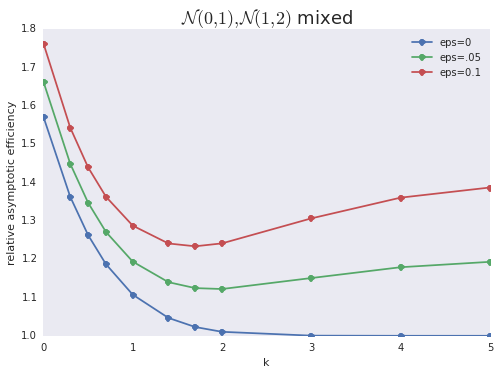In :
asympt_var_case2 = closure_variance((0,0),(1,10)) # case 1 with N(0,1) + N(0,10)

In :
fig2,ax=subplots()
ax.plot(kvals,[asympt_var_case2(k,0) for k in kvals],'-o',label='eps=0')
ax.plot(kvals,[asympt_var_case2(k,.05) for k in kvals],'-o',label='eps=.05')
ax.plot(kvals,[asympt_var_case2(k,.1) for k in kvals],'-o',label='eps=0.1')
ax.set_xlabel("k")
ax.set_ylabel("relative asymptotic efficiency ")
ax.legend(loc=0)
ax.set_title(r"$\mathcal{N}(0,1) , \mathcal{N}(0,10)$ mixed",fontsize=18)
ax.grid()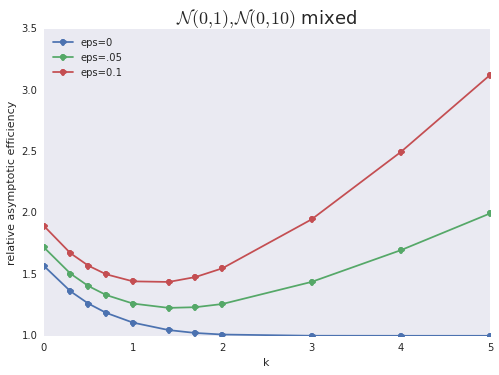In :
fig.get_axes().axis(ymax=3.5)
fig

Out: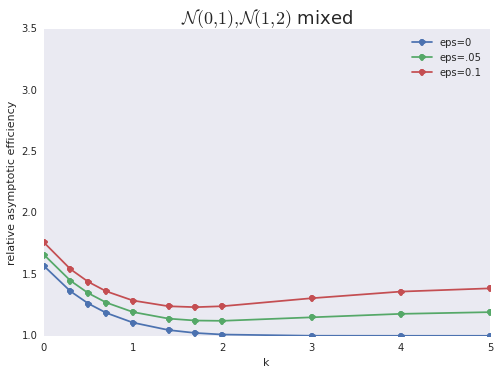## Computable Example¶

In :
nsamples = 200
xs = np.array([dual_set[bias_coin_gen.next()].rvs() for i in range(200)*nsamples ]).reshape(nsamples,-1)

In :
fig,ax=subplots()
ax.hist(np.mean(xs,0),20,alpha=0.8,label = 'mean')
ax.hist(np.median(xs,0),20,alpha=0.3,label ='median')
ax.legend()

Out:
<matplotlib.legend.Legend at 0x12d21970>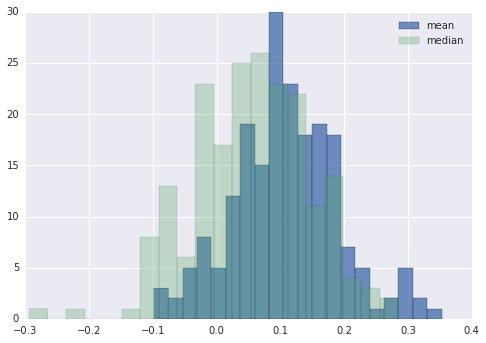• Maronna, R. A., R. D. Martin, and V. J. Yohai. "Robust Statistics: Theory and Methods". 2006.
In :
fig,ax=subplots()
sns.violinplot(np.vstack([np.median(xs,axis=0),np.mean(xs,axis=0)]).T,ax=ax,names=['median','mean']);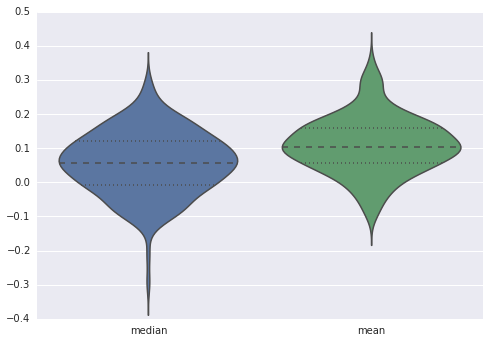In :
mma.push('data',xs[:,0].tolist())
mma.eval('psi[k_] := Function[x, Piecewise[{{x, Abs[x] < k}, {k*Sign[x], Abs[x] > k}}]]')

In :
def psi_est(k,x):
if x.ndim ==2 : # loop over columns
out = []
for i in range(x.shape):
data = x[:,i]
out.append( psi_est(k,data) ) # recurse
return np.array(out)
else:
mma.push('data',x.tolist())
return float(mma.eval('\[Mu] /. FindRoot[Plus @@ (psi[%d] /@ (data - \[Mu])) == 0, {\[Mu], 0}]'%(k)))

In :
hist(psi_est(1,xs),alpha=.7,label='k=1')
hist(psi_est(2,xs),alpha=.7,label='k=2')
hist(psi_est(3,xs),alpha=.7,label='k=3')
legend(loc=0)

Out:
<matplotlib.legend.Legend at 0x1313f750>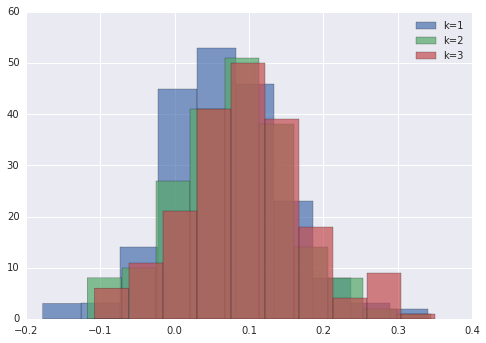In :
huber_est={k:psi_est(k,xs) for k in [1,1.5,2,3]}
huber_est = np.median(xs,axis=0)
huber_est = np.mean(xs,axis=0)

In :
fig,ax=subplots()
sns.violinplot(pd.DataFrame(huber_est),ax=ax)
ax.set_xticklabels(['median','1','1.5','2','3','mean']);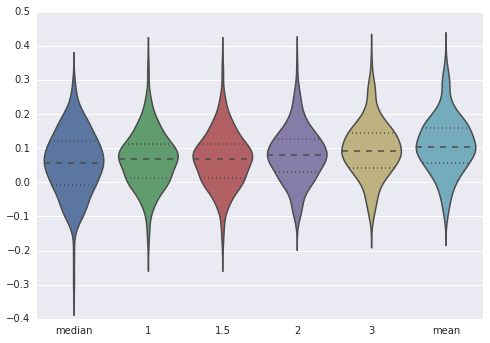In :
from sympy import mpmath, symbols, diff, Piecewise, sign, lambdify
from sympy.stats import density, cdf, Normal
from sympy.abc import k,x

In :
eps = symbols('epsilon')
lpdf=diff(cdf(Normal('x',0,1))(x)*(1-eps)+ eps*cdf(Normal('x',0,10))(x),x)
p = Piecewise((x,abs(x)<k),(k*sign(x),True))

In :
def asymptotic_variance(kval,epsval):
return float(numer/denom)

In :
asymptotic_variance(1,.05)

Out:
1.292336985078515
In :
asympt_var_case2(1.0001,.05)

Out:
1.2625286610649238
In :
def closure_on_asymptotic_variance(mn=(0,0),std=(1,10)):
from sympy.abc import k,x
eps = symbols('epsilon')
lpdf=diff(cdf(Normal('x',mn,std))(x)*(1-eps)+ eps*cdf(Normal('x',mn,std))(x),x)
def asymptotic_variance(kval,epsval):
p = Piecewise((x,abs(x)<kval),(kval*sign(x),True))
return float(numer/denom)
return asymptotic_variance

In :
case2=closure_on_asymptotic_variance()

In :
print case2(1.0001,.05)
print asympt_var_case2(1.0001,.05)

1.26296063799
1.26252866106

In :
fig2,ax=subplots()
ax.plot(kvals,[case2(k,0) for k in kvals],'-o',label='eps=0')
ax.plot(kvals,[case2(k,.05) for k in kvals],'-o',label='eps=.05')
ax.plot(kvals,[case2(k,.1) for k in kvals],'-o',label='eps=0.1')
ax.plot(kvals,[asympt_var_case2(k,0) for k in kvals],'--o',label='mma eps=0')
ax.plot(kvals,[asympt_var_case2(k,.05) for k in kvals],'--o',label='mma eps=.05')
ax.plot(kvals,[asympt_var_case2(k,.1) for k in kvals],'--o',label='mma eps=0.1')
ax.set_xlabel("k")
ax.set_ylabel("relative asymptotic efficiency ")
ax.legend(loc=0)
ax.set_title(r"$\mathcal{N}(0,1) , \mathcal{N}(0,10)$ mixed",fontsize=18)
ax.grid()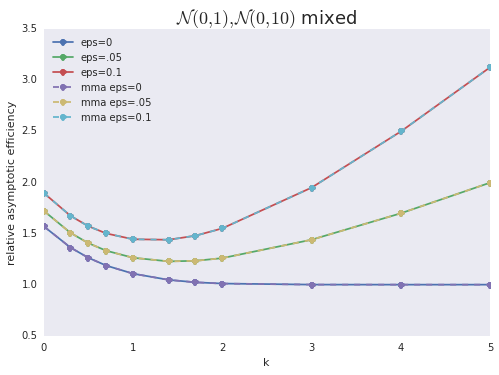In [ ]:

`